Vibroengineering PROCEDIA

Published: 13 December 2018

# Helicopter landing gear vibration analysis using Lagrange method

S. Sivakumar1
1SRM Institute of Science and Technology, Chennai, India
Views 372

#### Abstract

In this paper, landing dynamics for a helicopter fitted with an oleo pneumatic landing gear in the front and tail gears has been analysed with a seven degree of freedom mathematical model. Dynamic equations have been developed using Lagrange principle to investigate the effects of vibrations on the structure during landing phase of the helicopter. The bounce, roll, pitch, yaw acceleration response of helicopter landing obtained by numerical simulation in Matlab/Simulink. The developed model by Lagrange method will be useful to measure the vibration levels on landing and taxing of helicopter with uncertainties. This approach is also helpful to optimize the stiffness and damping properties of landing gear.

## 1. Introduction

Landing is the most critical operational phase of a helicopter. During landing of helicopter on the uneven surface, a large amplitude of vibrations transmitted to the helicopter fuselage structure causing safety and comfort problems to crew and passengers. Moreover, the helicopter also involves complex dynamics of main rotor, tail rotor, engine transmission system and flying dynamics. Landing gear plays an important role in absorbing the shocks and vibrations due to touch down impact and rolling on the deck or un even surface. So, the performance efficiency of the shock absorber is very much essential to meet the variable damping requirements to cope with varying operating conditions. Peter J. F. O. Reilly  presented an analytical model for dynamic analysis of landing and taking off of the aircraft from a moving deck. Fu Shang et al.  derived the SH-2Fship board dynamic model to prescribe the safe environment for taking off and landing of a helicopter from the flight deck. In his study, energy method used for deriving equations of motion for ship board dynamic motion and assumptions to develop a dynamic model of helicopter. Bernard  studied analytic approach of helicopter ship testing simulation for an EH101 helicopter and CPF ship model. Black well and Feik  have described a mathematical model of a helicopter landing on an arbitrarily moving deck. The mathematical model of two main oleo and single tail oleo developed and analyzed for oleo load and oleo deflection for deck landing. Kim and Tilbury  presented a six degree of freedom mathematical model for a helicopter to investigate the combined action between fly bar and the main rotor blades make it easier for a pilot to fly. Li pan and Chen Renliang  presented a helicopter comprehensive analysis by developing a mathematical model with the feature of flexibility and mathematical simplicity. Tulio Salazar  developed a mathematical model for a helicopter with a main rotor hub and tail rotor configuration to simulate flying behavior of the helicopter. An active landing gear behavior was demonstrated by Sivakumar and Haran  by developing six degree of freedom mathematical model of full aircraft with tricycle landing gear configuration. The author has investigated non-linear analysis of oleo pneumatic landing gear in . Most of the papers have shown the two degree of freedom mathematical model of single landing gear. This model does not include pitch, roll and yaw degrees-of-freedom. The effects of longitudinal and lateral interconnections cannot be studied in the two degrees-of-freedom system model. In this study, the seven degree of freedom math’s model of helicopter with landing gear has been developed using Lagrange method for landing dynamics of helicopter.

## 2. Mathematical model formulation

The full helicopter dynamic model built by considering typical US-Navy sea hawk helicopter version. The helicopter fuselage body or concentrated mass $M$ is free to bounce, roll, pitch and yaw. The fuselage mass $M$ is connected to the three lumped masses, ${m}_{l}$ and ${m}_{r}$ which are tail, front left and front right landing gears piston and tire viewed from front. The front landing gears are called left and right main landing gears and the rear one is called tail landing gear. They are free to bounce vertically with respect to the fuselage mass. The full helicopter model contains four degrees-of-freedom for the helicopter fuselage mass (bounce, roll, pitch, yaw), and three degrees-of freedom for the vertical motions of the tail landing gear tire mass and for the front main landing gears tire masses. In this work, the seven degrees-of-freedom vibration model of the full helicopter as indicated in figure1.This model represents bounce, pitch, roll and yaw motion of the helicopter as $x$, $\alpha$, $\gamma$, $\phi$ about the axes respectively. The displacements and ground excitations of the left, right and tail main landing gears as ${x}_{1}$, ${x}_{2}$, ${x}_{3}$ and, ${y}_{2}$, ${y}_{3}$.

The stiffness and damping constants of left shock strut, right and tail gear shock struts indicated as, ${k}_{2}$, ${k}_{3}$ and ${G}_{1}$, ${G}_{2}$, The stiffness and damping constants of left gear tire, right and tail gear tires is denoted as ${c}_{1}$, ${c}_{2}$, ${c}_{3}$. and ${G}_{x}$, ${G}_{y}$, ${G}_{z}$.The tail landing gear is located at a distance of $d$ from centre of gravity (C.G) position. The main landing gears positioned at $e$-distance from C.G. $b$-distance from C.G to left main landing gear. $a$-distance from C.G to right main landing gear. The dynamic equations have been derived by considering $x$, $\phi$, $\alpha$, for bounce, yaw motion along vertical axis, pitch about lateral axis and roll about longitudinal axis. In this study, helicopter aerodynamic forces and rotor thrust are not taken in to account. In this work, the helicopter landing dynamics equations are written by using Lagrange principle from the model as in Eqs. (1)-(15).

Fig. 1Mathematical model of helicopter with landing gears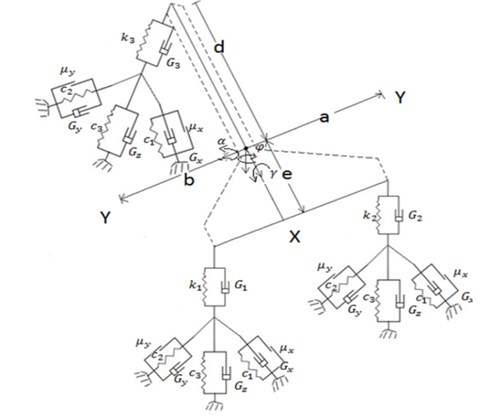Total kinetic energy ($K$) is calculated as:

1
$K=\frac{1}{2}m{\stackrel{˙}{x}}^{2}+\frac{1}{2}{I}_{xx}{\stackrel{˙}{\gamma }}^{2}+\frac{1}{2}{I}_{yy}{\stackrel{˙}{\alpha }}^{2}+\frac{1}{2}{I}_{zz}{\stackrel{˙}{\phi }}^{2}+\frac{1}{2}{m}_{l}{{\stackrel{˙}{x}}_{1}}^{2}+\frac{1}{2}{m}_{r}{{\stackrel{˙}{x}}_{2}}^{2}+\frac{1}{2}{m}_{t}{{\stackrel{˙}{x}}_{3}}^{2}.$

Total potential energy ($U$) is calculated as:

2
$U=\frac{1}{2}{k}_{1}{\left(x-{x}_{1}-b\gamma -e\alpha +h\phi \right)}^{2}+\frac{1}{2}{k}_{2}{\left(x-{x}_{2}-a\gamma -e\alpha +h\phi \right)}^{2}+\frac{1}{2}{k}_{3}{\left(x-{x}_{3}+d\alpha +h\gamma +j\phi \right)}^{2}+\frac{1}{2}{c}_{3}\left({x}_{1}-{y}_{1}\right)+\frac{1}{2}{c}_{2}{\left({x}_{1}\mathrm{c}\mathrm{o}\mathrm{s}\theta -{y}_{1}\mathrm{c}\mathrm{o}\mathrm{s}\theta \right)}^{2}+\frac{1}{2}{c}_{1}{\left({x}_{1}\mathrm{c}\mathrm{o}\mathrm{s}\theta -{y}_{1}\mathrm{c}\mathrm{o}\mathrm{s}\theta \right)}^{2}+\frac{1}{2}{c}_{3}{\left({x}_{2}-{y}_{2}\right)}^{2}+\frac{1}{2}{c}_{2}{\left({x}_{2}\mathrm{c}\mathrm{o}\mathrm{s}\theta -{y}_{2}\mathrm{c}\mathrm{o}\mathrm{s}\theta \right)}^{2}+\frac{1}{2}{c}_{1}{\left({x}_{2}\mathrm{c}\mathrm{o}\mathrm{s}\theta -{y}_{2}\mathrm{c}\mathrm{o}\mathrm{s}\theta \right)}^{2}+\frac{1}{2}{c}_{3}{\left({x}_{3}-{y}_{3}\right)}^{2}+\frac{1}{2}{c}_{2}{\left({x}_{3}\mathrm{c}\mathrm{o}\mathrm{s}\theta -{y}_{3}\mathrm{c}\mathrm{o}\mathrm{s}\theta \right)}^{2}+\frac{1}{2}{c}_{1}{\left({x}_{3}\mathrm{c}\mathrm{o}\mathrm{s}\theta -{y}_{3}\mathrm{c}\mathrm{o}\mathrm{s}\theta \right)}^{2}+{\mu }_{x}{R}_{N}\left({x}_{1}\mathrm{c}\mathrm{o}\mathrm{s}\theta -{y}_{1}\mathrm{c}\mathrm{o}\mathrm{s}\theta \right)+{\mu }_{y}{R}_{N}\left({x}_{1}\mathrm{c}\mathrm{o}\mathrm{s}\theta -{y}_{1}\mathrm{c}\mathrm{o}\mathrm{s}\theta \right)+{\mu }_{x}{R}_{N}\left({x}_{2}\mathrm{c}\mathrm{o}\mathrm{s}\theta -{y}_{2}\mathrm{c}\mathrm{o}\mathrm{s}\theta \right)+{\mu }_{y}{R}_{N}\left({x}_{2}\mathrm{c}\mathrm{o}\mathrm{s}\theta -{y}_{2}\mathrm{c}\mathrm{o}\mathrm{s}\theta \right)+{\mu }_{x}{R}_{N}\left({x}_{3}\mathrm{c}\mathrm{o}\mathrm{s}\theta -{y}_{3}\mathrm{c}\mathrm{o}\mathrm{s}\theta \right)+{\mu }_{y}{R}_{N}\left({x}_{3}\mathrm{c}\mathrm{o}\mathrm{s}\theta -{y}_{3}\mathrm{c}\mathrm{o}\mathrm{s}\theta \right).$

Total Dissipative energy ($D$) is calculated as:

3

By applying Lagrange method:

4
$\frac{d}{dt}\left(\frac{\partial K}{\partial {\stackrel{˙}{q}}_{k}}\right)-\frac{\partial K}{\partial {q}_{k}}+\frac{\partial D}{\partial {\stackrel{˙}{q}}_{k}}+\frac{\partial U}{\partial {q}_{k}}={Q}_{k},$
${q}_{k}=f\left(x,{x}_{1},{x}_{2},{x}_{3},\gamma ,\alpha ,\phi ,{y}_{1},{y}_{2},{y}_{3}\right),$
${\stackrel{˙}{q}}_{k}=f\left(\stackrel{˙}{x},{\stackrel{˙}{x}}_{1,}{\stackrel{˙}{x}}_{2},{\stackrel{˙}{x}}_{3},\stackrel{˙}{\gamma },\stackrel{˙}{\alpha },\stackrel{˙}{\phi },{\stackrel{˙}{y}}_{1},{\stackrel{˙}{y}}_{2},{\stackrel{˙}{y}}_{3}\right),$
5
$\frac{d}{dt}\left(\frac{\partial K}{\partial {\stackrel{˙}{q}}_{k}}\right)=m\stackrel{¨}{x}+{I}_{xx}\stackrel{¨}{\gamma }+{I}_{yy}\stackrel{¨}{\alpha }+{I}_{zz}\stackrel{¨}{\phi }+{m}_{l}{\stackrel{¨}{x}}_{1}+{m}_{r}{\stackrel{¨}{x}}_{2}+{m}_{t}{\stackrel{¨}{x}}_{3},$
6
$\frac{\partial D}{\partial {q}_{k}}=-{G}_{1}b\stackrel{˙}{x}+{G}_{1}b{\stackrel{˙}{x}}_{1}+{G}_{1}{b}^{2}\stackrel{˙}{\gamma }+{G}_{1}be\stackrel{˙}{\alpha }-{G}_{1}bh\stackrel{˙}{\phi }-{G}_{1}\stackrel{˙}{x}e+{G}_{1}{\stackrel{˙}{x}}_{1}e+{G}_{1}be\stackrel{˙}{\gamma }$

7
$\frac{\partial U}{\partial {q}_{k}}=-{k}_{1}bx+{k}_{1}b{x}_{1}+{k}_{1}{b}^{2}\gamma +{k}_{1}be\alpha -{k}_{1}bh\phi -{k}_{1}xe+{k}_{1}{x}_{1}e+{k}_{1}be\gamma -{k}_{1}he\phi$

The matrix formulation is written as (8):

8
$M\stackrel{¨}{X}+C\stackrel{˙}{X}+KX=0,$

where:

${S}_{1}=\stackrel{˙}{x}\left(-{G}_{1}b-{G}_{1}e+{G}_{1}h+{G}_{2}a-{G}_{2}e+{G}_{2}h-{G}_{3}d+{G}_{3}h+{G}_{3}r\right),$
${S}_{2}=\stackrel{˙}{\gamma }\left({G}_{1}{b}^{2}+{G}_{1}be-{G}_{1}bh+{G}_{2}{a}^{2}-{G}_{2}ae+{G}_{2}ah-{G}_{3}dh+{G}_{3}{h}^{2}+{G}_{3}rh\right),$
${S}_{3}=\stackrel{˙}{\alpha }\left({G}_{1}be+{G}_{1}{e}^{2}-{G}_{1}eh-{G}_{2}ae+{G}_{2}{e}^{2}-{G}_{2}eh-{G}_{3}d+{G}_{3}{d}^{2}+{G}_{3}dh\right),$
${S}_{4}=\stackrel{˙}{\phi }\left(-{G}_{1}bh-{G}_{1}eh+{G}_{1}{h}^{2}+{G}_{2}ah-{G}_{2}eh+{G}_{2}{h}^{2}+{G}_{3}dr+{G}_{3}rh+{G}_{3}{r}^{2}\right),$

${T}_{1}=x\left(-{k}_{1}b-{k}_{1}e+{k}_{1}h+{k}_{2}a-{k}_{2}e+{k}_{2}h-{k}_{3}d+{k}_{3}h+{k}_{3}r\right),$
${T}_{2}=\gamma \left({k}_{1}{b}^{2}+{k}_{1}be-{k}_{1}bh-{k}_{2}ae+{k}_{2}ah+{k}_{2}{a}^{2}+{k}_{3}{h}^{2}+{k}_{3}rh+{k}_{3}dh\right),$
${T}_{3}=\alpha \left(+{k}_{1}be+{k}_{1}{e}^{2}-{k}_{1}eh-{k}_{2}ae+{k}_{2}{e}^{2}-{k}_{2}eh+{k}_{3}{d}^{2}+{k}_{3}dh+{k}_{3}dr\right),$
${T}_{4}=\phi \left(-{k}_{1}bh-{k}_{1}he+{k}_{1}{h}^{2}+{k}_{2}ah-{k}_{2}eh+{k}_{2}{h}^{2}+{k}_{3}dr+{k}_{3}rh+{k}_{3}{r}^{2}\right),$

The Eqs. (9)-(15) can be solved separately in Matlab/Simulink as:

9
$m\stackrel{¨}{x}+{S}_{1}\stackrel{˙}{x}+{T}_{1}x=0,$
10
${I}_{xx}\stackrel{¨}{\gamma }+{S}_{2}\stackrel{˙}{\gamma }+{T}_{2}\gamma =0,$
11
${I}_{yy}\stackrel{¨}{\alpha }+{S}_{3}\stackrel{˙}{\alpha }+{T}_{3}\alpha =0,$
12
${I}_{zz}\stackrel{¨}{\phi }+{S}_{4}\stackrel{˙}{\phi }+{T}_{4}\phi =0,$
13
${m}_{l}{\stackrel{¨}{x}}_{1}+{S}_{5}{\stackrel{˙}{x}}_{1}+{T}_{5}{x}_{1}=0,$
14
${m}_{r}{\stackrel{¨}{x}}_{2}+{S}_{6}{\stackrel{˙}{x}}_{2}+{T}_{6}{x}_{2}=0,$
15
${m}_{t}{\stackrel{¨}{x}}_{3}+{S}_{7}{\stackrel{˙}{x}}_{3}+{T}_{7}{x}_{3}=0.$

## 3. Landing simulation

The Simulink model of typical helicopter with landing gear system has been developed from the dynamic equations written in Section 2 as shown in Fig. 2. Several trials of numerical simulations can be done by using this Simulink model set up. The helicopter of fuselage mass 9000 kg is considered for numerical simulation. The helicopter and landing gear parameters used for numerical simulation has taken from Blackwell and Feik  tabulated in Table1 and Table 2.

As per FAR 23.725, recommended free drop height range for both MLG and NLG is minimum 9.2 in and maximum 18.7 in. In this simulation, the higher value of 0.4 m is taken for landing. simulation in Matlab/Simulink environment and landing gear touches the runway at 2.5 s on even ground. During landing simulation both the main landing gears touch first and the tail landing gear later. The vertical acceleration, pitch, roll, yaw acceleration responses due to touch down impact of the main landing gear in time domain is presented in the Fig. 3-6.

Fig. 2Simulation set up in Matlab/Simulink environment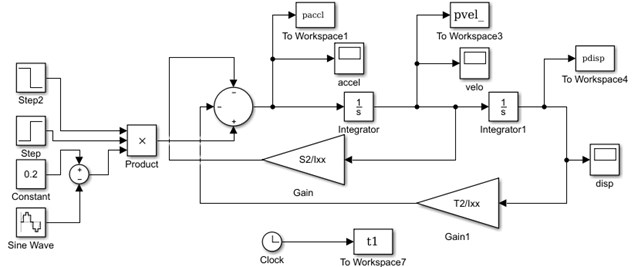Fig. 3Bounce acceleration response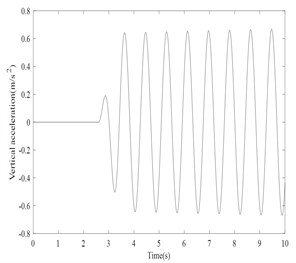Fig. 4Roll acceleration response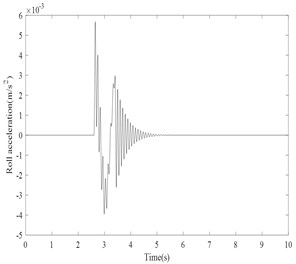Fig. 5Pitch acceleration response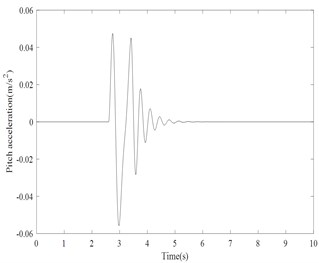Fig. 6Yaw acceleration response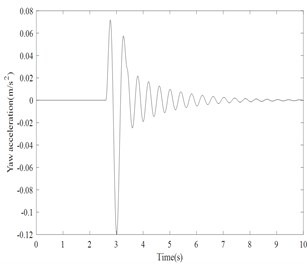Results shows that vertical acceleration is not settled within a short time. The bounce, roll, pitch and yaw acceleration levels are damped within 2 to 4 secs. The settling time is very less for the helicopter to become stable. The trend of the results for a typical simulation is qualitatively matching with the reference given in . It is very difficult to do the real time experimental validation with the typical helicopter. In future study, the typical helicopter has been developed in other Multi body dynamics software’s like ADAMS or LMS to do landing dynamics.

Table 1Helicopter parameters

 Description Symbol Value Units Helicopter fuselage mass $M$ 9000 kg Mass moment of inertia about $XX$ axis ${I}_{xx}$ 9.0e3 kg.m² Mass moment of inertia about $YY$ axis ${I}_{yy}$ 6.3e4 kg.m² Mass moment of inertia about $ZZ$ axis ${I}_{zz}$ 6.0e4 kg.m² Distance from C.G to tail gear $d$ 3.3 m Distance from C.G to horizontal axis of main gear $e$ 1.5 m Distance from C.G to left main landing gear $a$ 1.4 m Distance from C.G to right main landing gear $b$ 1.4 m

Table 2Helicopter landing gear parameters

 Parameter Main gear Tail gear Units Parameter Main gear Tail gear Units ${k}_{1}$ 3.6e5 – N/m ${c}_{2}$ 4.5e5 6.0e5 N/m ${k}_{2}$ 5.0e6 – N/m ${c}_{3}$ 1.2e6 1.0e6 N/m ${k}_{3}$ – 2.0e6 N/m ${G}_{x}$ 2.0e4 2.0e4 N.s/m ${G}_{1}$ 2.2e4 – N.s/m ${G}_{y}$ 2.0e4 2.0e4 N.s/m ${G}_{2}$ 4.0e4 – N.s/m ${G}_{z}$ 1.0e4 1.0e4 N.s/m ${G}_{3}$ – 3.0e4 N.s/m ${\mu }_{x}$ 0.7 0.7 – ${c}_{1}$ 1.4e6 8.0e5 N/m ${\mu }_{y}$ 0.7 0.7 –

## 4. Conclusions

In this research work, helicopter with landing gear seven degree of freedom mathematical model using Lagrange method has been developed to investigate dynamic response. The response of bounce, pitch, roll, yaw accelerations are obtained to verify the developed model. The responses are stable. This numerical simulation procedure is helpful to check the vibration levels of aircraft during landing and taxing on the various ground uncertainties. By using this model, the stiffness and damping coefficient of landing gear component obtained experimentally during drop test can be evaluated theoretically. This procedure enables the designer to optimize the design parameters of landing gear in the initial design stage itself for achieving better performance of landing gears.

#### Cited by

Blood Product Supply for a Helicopter Emergency Medical Service